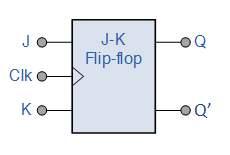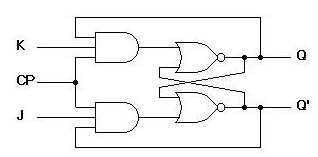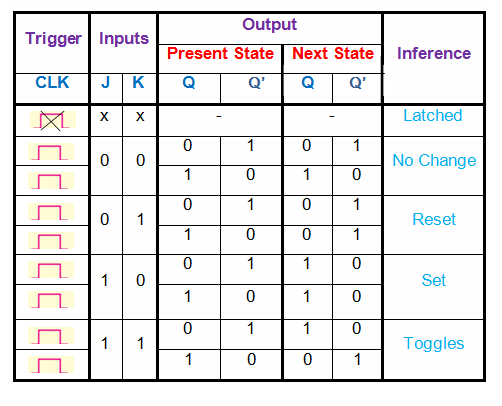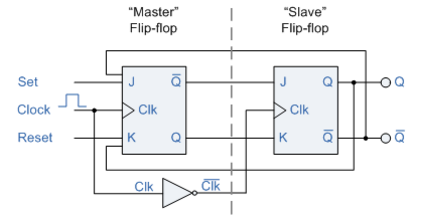# JK Flip Flop Truth Table and Circuit Diagram

Before we learn what a JK flip flop is, it would be wise to learn what, actually, a flip flop is. A flip-flop is a bistable circuit made up of logic gates.

A bistable circuit can exist in either of two stable states indefinitely and can be made to change its state by means of some external signal.

The most important use of this property is that a flip flop can “store” binary information.

We have seen that a logic gate can make a logical decision based on the immediate conditions at the input terminals. However, the gates normally do not have a memory characteristic to retain the input data.

On the other hand, flip flops have the valuable feature of remembering. The reason is that a flip-flop circuit is bistable.

Because the flip-flop’s output remains at a 0 or 1 depending on the last input signal, the flip-flop can be said to “remember”. Another name for the flip-flop is bistable multivibrator.

We shall discuss the most important type of flip-flops i.e.  JK flip flop in this post.

## JK Flip Flop Circuit Diagram

The JK flip-flop is probably the most widely used and is considered the universal flip-flop because it can be used in many ways.

In our previous article we discussed about the S-R Flip-Flop . Actually,  a J-K Flip-flop  is a modified version of an S-R flip-flop with no “invalid”  output state . And this is achieved by  the addition of a clock input circuitry with the SR flip-flop which prevents the  “invalid “output condition that can occur when both inputs S and R are equal to logic level “1”.

The logic symbol for the JK flip-flop is illustrated in Fig. 1.Fig.1 : Logic Symbol for JK flip-flop

The inputs labeled J and K are the data inputs ( which used to be S and R inputs in S-R Flip-flop).

The input labeled CLK is the clock input.

Outputs Q and Q’ are the usual normal and complementary outputs .

The circuit diagram of the J-K Flip-flop is shown in fig.2 .Fig.2

The old two-input AND gates of the S-R flip-flop have been replaced with 3-input AND gates .And the third input of each gate receives feedback from the Q and Q’ outputs.

Now from the above diagram it is clear that, this allows the J input to have effect only when the circuit is reset, i.e. Q=0 and Q’ =1 . And permit the K input to have effect only when the circuit is set i.e. Q=1 and Q’ =0.

In other words, the two inputs are interlocked, so that they cannot both be activated simultaneously.

If the circuit is “set,” the J input is inhibited by the 0 status of Q’ through the lower AND gate; if the circuit is “reset,” the K input is inhibited by the 0 status of Q through the upper AND gate.

Now what happens when both J and K inputs are 1 !!!!!

Because of the selective inhibiting action of those 3-input AND gates, a “set” state inhibits input J so that the flip-flop acts as if J=0 while K=1 when in fact both are 1.

On the next clock pulse, the outputs will switch  or “toggle” from set (Q=1 and Q’=0) to reset (Q=0 and Q’=1). Conversely, a “reset” state inhibits input K so that the flip-flop acts as if J=1 and K=0 when in fact both are 1.

Then the next clock pulse toggles the circuit again from reset to set.

### JK Flip Flop Truth Table

The truth table of a JK  flip flop is shown below.Table.1

This table shows four useful modes of operation.

When J = K = 0 and clk = 1; output of  both AND gates will be 0; when any one input of NOR gate is 0 output of NOR gate will be complement of other input, so output remains as previous output or we can say the flip-flop is in the hold (or disabled) mode. In the hold mode, the data inputs have no effect on the outputs.The outputs “hold” the last data present.

When J =0  K =1 and clk = 1; output of AND gate connected to K will be Q and corresponding NOR gate output will be 0; which RESETs the flipflop.

When J =1  K = 0 and clk = 1; output of AND gate connected to J will be Q’ and corresponding NOR gate output will be 0; which the SETs the flipflop.

When J=1  K = 1 and clk = 1;, repeated clock pulses cause the output to turn off-on-off-on-off-on and so on. This off-on action is like a toggle switch and is called toggling. Each clock pulse toggles the outputs to switch to their opposite states.

### Race Around Condition In JK Flip-flop

For J-K flip-flop, if J=K=1, and if clk=1 for a long period of time, then Q output will toggle as long as CLK is high, which makes the output of the flip-flop unstable or uncertain. This problem is called race around condition in J-K flip-flop.

This problem can be avoided by ensuring that the  clock input is at logic “1” only for a very short time.This introduced the concept of Master Slave JK flip flop.

### Master-Slave JK Flip-Flop

Master-slave J-K flip flop is designed using two J-K flipflops connected in  cascade. Out of these, one acts as the master and receives the  external inputs and the other acts as a slave and takes its inputs  directly from the master flip-flop . The figure of a master-slave J-K flip flop is shown below.Fig.3

From the above figure we can see that both the J-K flip flops are presented in a series connection.

The output of the master J-K flip flop is fed to the input of the slave J-K flip flop.

The output of the slave J-K flip flop is given as a feedback to the input of the master J-K flip flop.

The clock pulse [Clk] is given to the master J-K flip flop and it is sent through a NOT Gate and thus inverted before passing it to the slave J-K flip flop.

### Operation

The input signals J and K are connected to the “Master” flip-flop which locks the input while the clock (Clk) input is high at logic level “1”.

As the clock input of the “Slave” flip-flop is the inverse (complement) of the “Master” clock input, the outputs from the “Master” flip-flop are only seen by the “Slave” flip-flop when the clock input goes “LOW” to logic level “0”.

Therefore on the “High-to-Low” transition of the clock pulse the locked outputs of the “Master” flip-flop are fed through to the JK inputs of the “Slave” flip-flop and thus making this type of flip-flop edge or pulse-triggered.

Then, the circuit accepts input data when the clock signal is “HIGH”, and passes the data to the output on the falling-edge of the clock signal.

In other words, the Master-Slave JK Flip-flop is a “Synchronous” device as it only passes data with the timing of the clock signal.